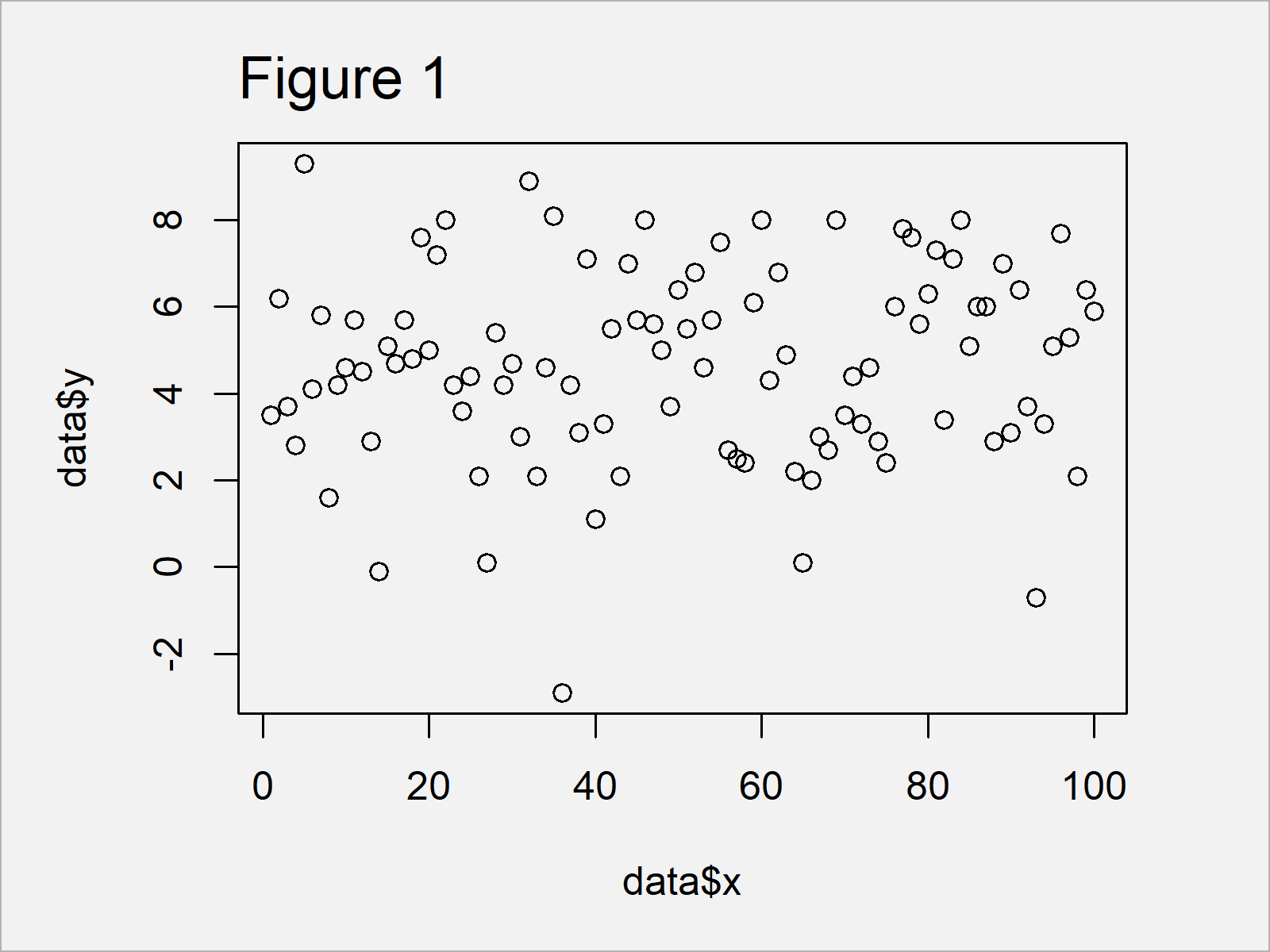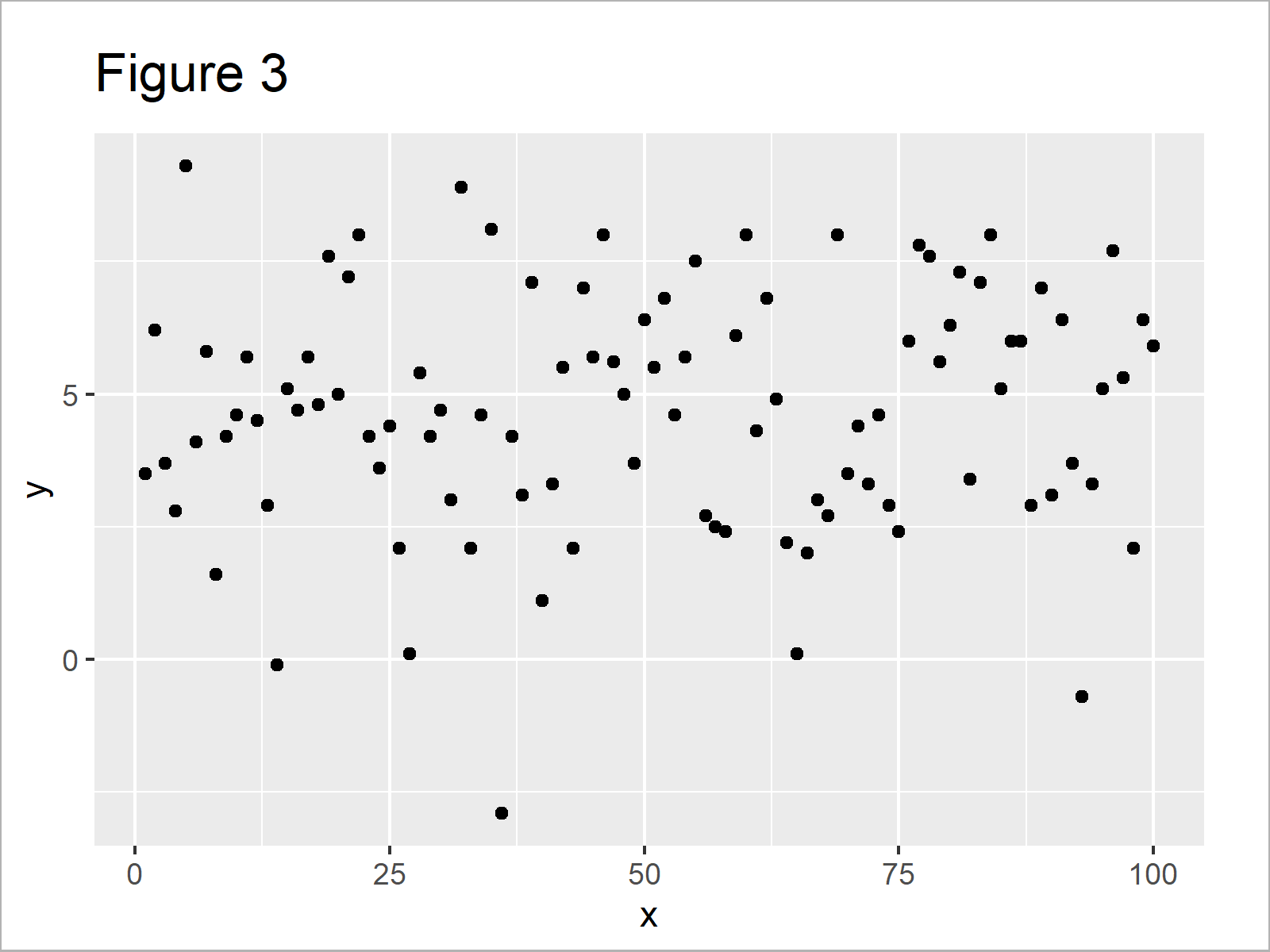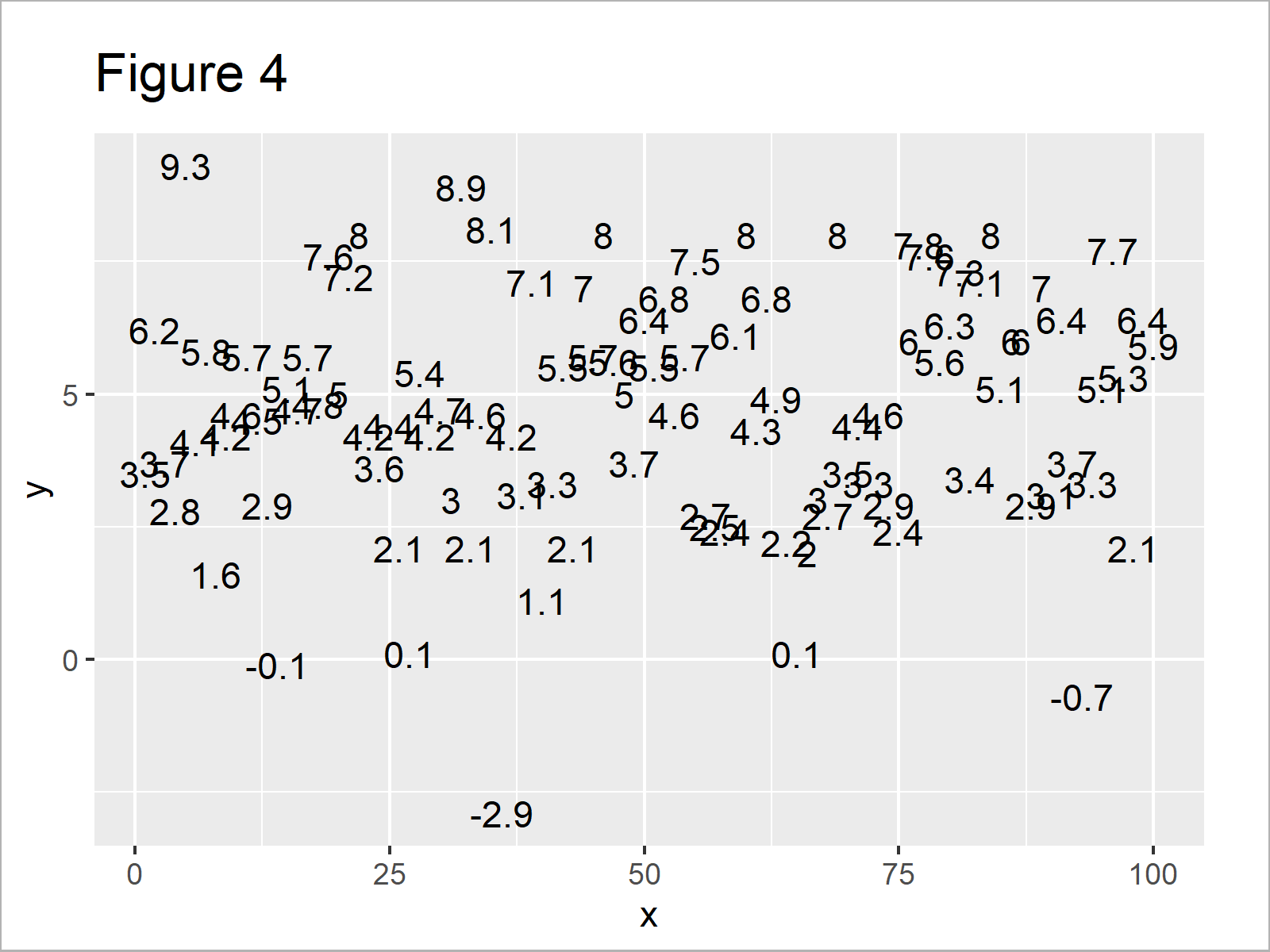# Display Only Values in Plot in R (2 Examples)

In this tutorial, I’ll demonstrate how to show only text labels in a scatterplot in the R programming language.

The content of the tutorial looks as follows:

Here’s how to do it.

## Example Data

The first step is to create some example data:

```set.seed(5639478)                       # Create example data
data <- data.frame(x = 1:100,
y = round(rnorm(100, 5, 2), 1))Table 1 reveals that our example data has the two columns “x” and “y”.

## Example 1: Draw Only Text Labels in Plot Using Base R

This example explains how to draw an xy-plot that shows only the values of our data using the basic installation of the R programming language.

First, let’s draw a regular scatterplot with points:

```plot(x = data\$x,                        # Default plot using Base R
y = data\$y)```In Figure 1 you can see that we have created a Base R plot with points.

If we want to replace these points by the values of our data, we can use the type argument of the plot function and the text function as shown below:

```plot(x = data\$x,                        # Plot with text using Base R
y = data\$y,
type = "n")
text(x = data\$x,
y = data\$y,
labels = data\$y)```The output of the previous R syntax is shown in Figure 2 – A scatterplot with values instead of points.

## Example 2: Draw Only Text Labels in Plot Using ggplot2 Package

This example demonstrates how to use the ggplot2 package to draw a plot with text labels only.

In order to use the commands and functions of the ggplot2 package, we first need to install and load ggplot2:

```install.packages("ggplot2")             # Install & load ggplot2 package
library("ggplot2")```

In the next step, we can draw a scatterplot with points:

```ggplot(data, aes(x, y, label = y)) +    # Default plot using ggplot2
geom_point()```After running the previous R programming syntax the ggplot2 scatterplot shown in Figure 3 has been created.

If we want to exchange the points of the previous scattershot, we simply have to use the geom_text function instead of the geom_point function:

```ggplot(data, aes(x, y, label = y)) +    # Plot with text using ggplot2
geom_text()```As visualized in Figure 4, we have plotted a ggplot2 graph with values instead of dots by executing the previous R programming code.

## Video & Further Resources

Do you want to know more about text labels and plots? Then you may want to watch the following video that I have published on my YouTube channel. I explain the topics of this article in the video:

Besides that, you might have a look at the related tutorials of this website.

In this tutorial you have learned how to display only the values of a scatterplot in the R programming language. Please let me know in the comments section, if you have any further questions.

Subscribe to the Statistics Globe Newsletter